# Python变量和对象类型速记手册

Python中关于变量和对象类型有一些重要的概念：

 变量不受类型约束 变量并不是一块内存空间的标签，只是对象的名字，是没有类型的，相当于一个可以指向任何对象void指针。类型属于对象，而不是变量。 动态类型 它自动地跟踪你的类型而不是要求声明代码；Python中没有类型声明，运行的表达式，决定了建立和使用的对象的类型 强类型 类型是不可变的，一旦发生强制类型转换，则意味着新的对象的建立。你只能对一个对象进行有效的操作。 多态 由于类型不受约束，Python编写的操作通常可以自动地适用于不同类型的对象，只要他们支持一种兼容的接口（类似C++里模板的概念），就像‘+’操作对于数字为加法，对于序列为合并。 对象头部信息 对象的内存空间，除了值外，还有 1 一个类型标识符（标识对象类型） 2 一个应用计数器（用来决定是不是可以回收这个对象）sys.getrefcount(1) 对象类型检测 在Python中，我们编写对象接口而不是类型。不关注与特定类型意味着代码会自动地适应于他们中的很多类型：任何具有兼容接口的对象均能工作。 1. type(L) == type([]) 2. type(L) == list 3. isinstance(L,list) 尽管支持类型检测，但这并不是一个“Python式”的思维方式，破坏了代码的灵活性。 赋值 基本赋值、元组赋值、列表赋值和多目标赋值。在赋值语句中，即使没有圆括号，也能识别出来这是一个元组。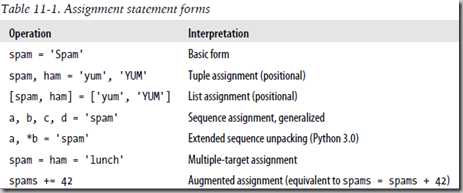序列赋值语句:支持右侧任何可迭代对象（左右元素数目相同） [a,b,c] = (1,2,3)或 [a,b,c] = '123'或 red,green,blue = range(3)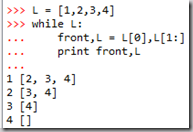交换值不需要中间变量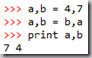需要注意引用的陷阱：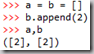对于支持原地修改的对象而言，增强赋值语句会执行原地修改运算L.append(4) L.extend([7,8])，比合并（复制过程）L = L +  执行得更快.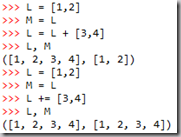隐式赋值语句：模块导入import,from;函数和类的定义def,class;for循环变量;函数参数 在函数中接收元组和列表 当要使函数接收元组或字典形式的参数的时候，有一种特殊的方法，它分别使用*和**前缀。这种方法在函数需要获取可变数量的参数的时候特别有用。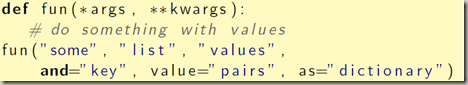引用传递 在 Python 中所有赋值操作都是“引用传递”。当把一个对象赋给一个数据结构元素或者变量名时，Python总是会存储对象的引用，而不是对象的一个拷贝（除非明确要求拷贝）。 通过将一些基本数据类型（数值、字符串、元组）设为不可改变对象，可以模拟“值传递”，例如： userName = ‘tonyseek’   #字符串对象为不可变对象，保护完整性 otherUserName = userName  #指向新创建的另一个字符串对象 otherUserName = ‘It’s not tonyseek’  #改变的不是userName指向的内容 存储对象引用 列表和元组都被认为是“对象引用”的数组(在标准Python解释器内部，就是C数组而不是链接结构，索引速度较快)。 浅拷贝和深拷贝 浅拷贝只拷贝顶层结构，深拷贝是递归的拷贝。 如果你想要复制一个列表或者类似的序列或者其他复杂的对象（不是如整数那样的简单对象 ），那么你可以使用切片操作符来取得拷贝。如果你只是想要使用另一个变量名，两个名称都 参考 同一个对象，那么如果你不小心的话，可能会引来各种麻烦。 X = [1,2,3] L1 = [X,X,X] L2 = [X[:],list(X),copy.copy()] #顶层复制 L.append(L) #无限循环对象，复合对象包含指向自身的引用· 不变对象的缓存和复用 作为一种优化，Python缓存了不变的对象并对其进行复用，例如小的整数和字符串。因为不能改变数字和字符串，所以无论对同一个对象有多少个引用都没有关系，从逻辑的角度看，这工作起来就像每一个表达式结果的值都是一个不同的对象，而每一个对象都是不同的内存。 垃圾收集 当最后一次引用对象后（例如，将这个变量用其他的值进行赋值），这个对象所有占用的内存空间将会自动清理掉

Python中有几种主要的核心数据类型：

## 1 数值【不可变】

 负数 -x 正数 +x 按位翻转 ~x=-(x+1) 绝对值 `abs(x)` 若x位复数，返回复数模 用x除以y，返回包含商和余数的tuple值(int(x/y),x%y) `divmod(x, y)` x的y次幕(x ** y ) x % modulo pow(x ,y [,modulo]) 返回值类型与x同 复数 `complex (x[,y])` 将x做实部，y做虚部创建复数 整数 `int(x)` 将字符串和数字转换为整数,对浮点进行舍位而非舍入 long(x) 将字符串和数字转换为长整形 浮点数 `float(x)` 将str和num转换为浮点对象 四舍五入，n为小数点位数 `round(x[,n])` 进制转换 hex(x) 将整数或长整数转换为十六进制字符串 oct(x) 将整数或长整数转换为八进制字符串 平方根 math.sqrt(math.pi*85) `>= x` 的最小整数 `math.ceil(x)` `<= x`的最大整数 `math.floor(x)` 对 `x` 朝向 0 取整 `math.trunc(x)` 随机：数字生成 >>> random.random()   #浮点数，x in the interval [0, 1) 0.8759095262051569 >>> random.getrandbits(16) #a python long int with k random bits 9213L >>> random.uniform(1,1000) #浮点数in the range [a, b) or [a, b] 400.88489201157114 >>> random.randint(1,1000) #整数random integer in range [a, b] 817 >>> random.randrange(0,1001,2) #整数from range([start,] stop[, step]) 822 随机：序列操作sequences --------- pick random element random sampling without replacement generate random permutation >>> random.choice([0,1,2,3]) #a random element 2 >>> random.sample(xrange(10000000), 3) #unique random elements [9435040, 5756107, 8741537] >>> random.shuffle(list)  #洗牌，就地shuffle list，无返回值.可选参数random是一个返回[0.0, 1.0)间浮点数的无参函数名，默认为random.random. On the real line, there are functions to compute uniform, normal (Gaussian), lognormal, negative exponential, gamma, and beta distributions. For generating distributions of angles, the von Mises distribution is available.
• 整型int
• 长整型long（无限精度）
• 浮点数float（双精度）
• 十进制数/小数decimal（固定精度浮点数）
• 复数complex
• 分数fraction

## 2 序列【有序】【索引：偏移量】

 序列通用型操作 内置函数或表达式 合并 ‘+’ 不允许+表达式中混合数字和字符串，使用'123'+str(9)或者int('123')+9 重复 ‘*’ >>> print '----------------------------------------' >>> print '-'*40 索引(dictionary使用键) seq[i]=seq[len(seq)-i]，i是偏移量 切片 seq[i:j:d]（步长d=-1表示分片将会从右至左进行，实际效果就是反转序列） 将列表片段重新赋值 s[i :j ] = r 删除列表中一个片段 del s[i :j ]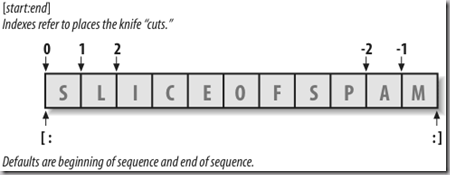求长度(dictionary,set支持) len(seq) 单个最大/最小值 max(seq),min(seq) 每项最大/最小值组成的序列 max(seq[,…]),min(seq[,…]) 迭代协议 列表解析，in成员关系测试，map内置函数以及sorted,sum,any,all调用等其他内置函数 sum,any,all sum返回可迭代对象中所有数字的和，可迭代对象中的任何/全部元素为True，any/all内置函数返回True 排序(dictionary返回键的列表,set支持，tuple不支持) sorted(seq) t = tuple(sorted(list(t))) 操作sorted(seq)返回对象；方法seq.sort()（tuple不支持）排序后不返回对象 从属关系(dictionary,set支持) x in seq, x not in seq 迭代 遍历访问元素：for x in seq: 遍历访问元素和索引：for index, item in enumerate(sequence): 使用range来产生索引：range(2,9,2) >>> range(0,10) [0, 1, 2, 3, 4, 5, 6, 7, 8, 9] >>> range(9,-1,-1) [9, 8, 7, 6, 5, 4, 3, 2, 1, 0] 同时遍历多个序列：zip(L1,L2)/map(None,L1,L2) zip函数转置二维列表： ```>>> l = [[1, 2, 3], [4, 5, 6]] >>> zip(*l) [(1, 4), (2, 5), (3, 6)]``` 列表解析(dictionary,set支持) 列表解析替代嵌套循环：for循环和列表解析（通常比对应的for循环运行得更快）都是通用迭代工具，都能工作于遵守迭代协议的任意对象（可遍历对象，包括可自逐行读取的文件） >>> M = [[1,2,3],[4,5,6],[7,8,9]] >>> column = [row for row in M if row%2 == 0] >>> column [2, 8] >>> diag = [M[i][i] for i in [0,1,2]] >>> diag [1, 5, 9] >>> squares = [x**2 for x in [1,2,3,4,5]] >>> squares [1, 4, 9, 16, 25] 列表解析也可用于元组的转换： >>> T = (1,2,3,4,5) >>> L = [x+20 for x in T] >>> L [21, 22, 23, 24, 25] >>> [line.upper() for line in open('script1.py')] map调用 >>> map(str.upper,open('script1.py')) #对可迭代对象每个元素都应用一个函数调用

### 2.1 字符串string【同构】【不可变】

 字符的有序集合(虽然元素间没有分隔符) >>> set('abcde') set(['a', 'c', 'b', 'e', 'd']) ‘\0’不会中断字符串 >>> s = "a\0b\tc\c" >>> s 'a\x00b\tc\\c' >>> len(s) 7 raw字符串 r’\temp\spam’ 类型方法 find(s),isdigit(),isalpha() upper(),lower(),replace(s0,s1),split(‘’),rstrip() 不可变：模拟改变元组 1 合并、分片、索引 where = S.find('SPAM') S = S[:where] + 'EGGS' +S[(where+4):] 2 字符串方法 S = S.replace('pl','pamal') #replace all S = S.replace('pl','pamal',1) #replace once 3 转化为可变对象 L = list(S) L = 'x' S = ''.jion(L) line = 'bob,hacker,40\n' line.rstrip() line.split(',') [bob,hacker,40] 4 字符串格式化 字符串格式化 s % type %[(name)][flags][width][.precision]code %[(键)][左对齐(-)正负号(+)补零(0)][整体宽度][.小数位数]code 基于元组的格式化：'%s is %d years old' % (name, age) 基于字典的格式化：'%s(age)d %(food)s' % vars() %s 任何对象的字符串表达(使用str) %r 任何对象的字符串表达(使用repr) %% 常量% 正则表达式（使用模式定义字符串） 实现更多搜索，分割，替换 >>> import re >>> match = re.match('[/]usr/(.*)/(.*)','/usr/home/jack') >>> type(match) >>> match.groups() ('home', 'jack') 对象的字符串表达 str(o):string used by print(user-friendly) 打印（用户友好） repr(o):as-code string used by echoes 交互式回显

### 2.2 unicode字符串 【同构】【不可变】 u’spam’

 支持所有的字符串操作

### 2.3 元组tuple【异构】【不可变】    t = (1,2,’3’)

 不可变 元组的不可变性只适用于元组本身的顶层结构而并非其内容： >>>T = (1,[2,3],4) >>> T = 'spam' TypeError: 'tuple' object does not support item assignment >>> T = ‘spam’ >>> T (1, ['spam', 3], 4) 类似“常数”声明：提供完整性约束 如果在程序中以元组的形式传递一个对象的集合，可以确保元组在程序中不会被另一个引用修改，而列表就没有这样的保证了。（利于编写大型程序） 与其他语言中的const概念不同，在Python中其是与对象相结合的（元组对象本身的不可变性），而不是变量。

### 2.4 列表list【异构】【可变】          l = [1,2,’3’]

 可变 禁止获取一个不存在的元素 禁止边界外的赋值 陷阱：遍历并修改列表 简单的for循环并不能修改列表，和C++中的迭代器用法不一样 `C++（for语句+迭代器）遍历并修改列表（输出222）` ```vector vint; vector::iterator i; vint.resize(3,1); for(i = vint.begin(); i != vint.end();++i) *i += 1; for(i = vint.begin(); i != vint.end();++i) cout << *i;``` `Python的for语句（这种foreach概念在C++中是没有的）不行：`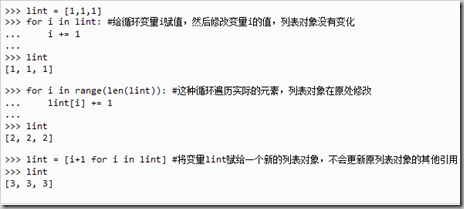类型方法 count(x),index(x) append(x),insert(i,x),extend(list) pop(i),remove(x),del L[i],del L[i:j] sort(),reverse() index(x)和remove(x)对匹配对象x的第一个元素进行操作，无匹配时异常

## 3 映射【无序】【索引：键】

### 3.1 字典dictionary【异构】【可变】 d = {1:’I’,2:’Love’,’3’:'Python’}

 可变 对新的字典的键赋值，会创建该键 `x[key] = value 可以删除一个键值对 禁止获取一个不存在的键值 x[key]` 键 键为不可变对象：数字和字符串，只包括(像数字和字符串这样的)不可变参数的元组，才可以作为字典中有效的键。 大多数Python对象可以作为键；但它们必须是可哈希的对象。像列表和字典这样的可变类型，由于它们不是可哈希的，所以不能作为键。 也有一些可变对象(很少)是可哈希的。 唯一 一键对应多个值是不允许的 获取元素列表 keys()返回键的列表,values()返回值的列表,items()返回tuples的列表 获取元素迭代器 iteritems(), iterkeys(), 和itervalues()这些函数与返回列表的对应方法相似，只是它们返回惰性赋值的迭代器，所以节省内存。 for k in d: 等价于 for k in d.keys(): '+'有序合并操作失效 update(x2) 用字典x2中的键/值对添加到原字典。重复键所对应的原有条目的值将被新键所对应的值所覆盖。 其他类型方法 get(x[,y]) 返回键x对应的值。若未找到返回default的值(注意，参数default的默认值为None)。get()方法和键查找(key-lookup)操作符( [ ] )相似，不同的是它允许你为不存在的键提供默认值。如果该键不存在，也未给出它的默认值，则返回None。此方法比采用键查找(key-lookup)更灵活，因为你不必担心因键不存在而引发异常。 pop(key) ,del d[key] clear() 删除词典的所有条目。 构造 D0 = {'name' = 'Bob', 'age' = 42} D1 = dict(name = 'Bob', age = 42) D2 = dict.fromkeys(seq, val=None) 创建并返回一个新字典，以seq中的元素做该字典的键，val做该字典中所有键对应的初始值(如果不提供此值，则默认为None) D3 = dict(zip(keylist,vallist)) 拷贝 copy() 浅拷贝：返回字典的高层结构的拷贝，但不复制嵌入结构，而复制那些结构的引用。 `copy模块：copy.copy(x) copy.deepcopy(x) ` 避免获取不存在的字典键错误 if d.has_keys(k)（最好使用if k in d）测试 get方法为不存在的键提供默认值 d.get(key,defaultval) D. get ( "Newton" , "unknown" ) # = ' unknown ' try:XXX except KeyError:XXX 语句捕获修复异常等

## 4 扩展

### 4.1 集合set【无序】【异构】【可变】s = set([1,2,’3’]) <=set(t|l|d)

 差集 ‘-’ 并集 ‘|’ 交集 ‘&’

### 4.2 文件file f = open(‘examples/data.txt’,’w’)

open参数 open(name[, mode[, buffering]]) -> file object

'r', 'w' or 'a' for reading (default),writing or appending. 写或追加模式下若文件不存在则创建它。

buffering参数：

for line in open('test.txt'):print line

 aString = input.read() aString = input.read(N) aString = input.readline() 读取[整个文件]到一个字符串 读取[之后N个字节]到一个字符串 读取[下一行（包括行末标志符）]到一个字符串 aList = input .readlines() aList = input.xreadlines() 读取[整个文件]到{字符串列表}

for line in open('test.txt').readlines():print line #比文件迭代器麻烦
while ... :   #效率比for差
line  = file.readline()
if not line:break

 output.write(aString) 写入{字节字符串}到文件，并不会添加行终止符 output.writelines(aList) 写入{列表内所有字符串}到文件

import sys
sys.stdout = open('log.txt', 'a') # Redirects prints to a file
...
print(x, y, x) # Shows up in log.txt

log = open('log.txt', 'a') # 2.6
print >> log, x, y, z # Print to a file-like object
print a, b, c # Print to original stdout

print >> sys.stderr, 'Bad'*8

 output.close() 手动关闭（当文件收集完成时会替你关闭文件） output.flush() 把输出缓冲区刷到硬盘上，但不关闭文件 anyFile.seek(N) 修改文件位置到偏移量N处（以便进行下一个操作）

1 eval能够吧字符串当做可执行代码
>>>eval['[1,2,3]']
[1,2,3]
2 pickle模块处理一般对象的存储
>>>import pickle
>>>pickle.dump(D,ofie)
>>>ofile.close()
>>>E = pickle.load(ifile)
3 struct模块处理文件中的二进制数据

### 4.4 布尔型bool

 0,'',(),[],{},None false 'string',>1,<-1 true

## 集合set

set（集合）是非常有用的数据类型，可以用来处理集合类型的数据。最开始在Python 2.3引入，从Python 2.4开始变为内置类型（不需导入模块）。

set支持一般的数学集合操作，处理较大的数据集合时是很方便的。

```>>>X = set("spam")    #make 2 sets out of sequences
```
```>>>Y = set(['h','a'])
```

```>>>Y.add('m')         #元素唯一性
```
```>>>Y.add('m')
```
```>>>X,Y
```
```(set(['a','p','s','m']),set(['h','a','m']))
```

```>>>X & Y              #Intersection 并集
```
```set(['a','m'])
```

```>>>X | Y              #Union 交集
```
```set(['a','p','s','h','m'])
```

```>>>X - Y              #Difference 差集
```
`set(['p','s'])`

```>>> a = {3:'c',2:'b',1:'a'}
```
```>>> print a
```
```{1: 'a', 2: 'b', 3: 'c'}
```
```>>> print sorted(a)
```
```[1, 2, 3]
```

```>>> b = set(a)
```
```>>> print b
```
```set([1, 2, 3])
```
```>>> print sorted(b)
```
```[1, 2, 3]
```
```
```
```>>> c = set(a.values())
```
```>>> print c
```
```set(['a', 'c', 'b'])
```
```>>> print sorted(c)
```
`['a', 'b', 'c']`

## 布尔型bool

Python2.3引入了明确的布尔数据类型bool，其值为True和False，而且True和False是预先定义的内置变量名。在内部，内置变量名True和False是bool的实例，实际上仅仅是内置的int的子类。

True和False的行为和整数1和0是一样的，除了他们有特定的逻辑打印形式（bool重新定义了str和repr的字符串格式)，也就是说，True仅仅是定制了显示格式的整数1，在Python中True+3=4！

## 占位符None

Python长期以为一直支持特殊的占位符对象None：

```>>> X = None       #None placeholder
```
```>>> X
```
```>>> print X
```
```None
```

```>>> L = [None]*10
```
```>>> L
```
```[None, None, None, None, None, None, None, None, None, None]
```

```>>> type(L)        #Types
```
```<type 'list'>
```
```>>> type(type(L))  #Even types are objects
```
`<type 'type'>`

## 十进制数decimal(固定精度浮点数)

`>>> import decimal`

```>>> d = 3.141
```
```>>> d + 1
```
```4.141
```
```>>> d + 1.111111
```
```4.252111
```
```>>> d + 1.1111111
```
```4.2521111000000005
```

```>>> d = decimal.Decimal('3.141')
```
```>>> d + 1
```
```Decimal('4.141')
```
```>>> d + 1.111111
```
```Traceback (most recent call last):
```
```  File "<console>", line 1, in <module>
```
```TypeError: unsupported operand type(s) for +: 'Decimal' and 'float'
```
```>>> d + decimal.Decimal('1.1111111')
```
```Decimal('4.2521111')
```

## 其他的Python要点

 性能优化 Python中一个主要的原则是：首先为了简单和可读性去编写代码，在程序运行后，并证明了确实有必要考虑性能后，再考虑该问题（time,timeit,profile模块）。更多情况是代码本身就已经足够快了。 面向对象 基本概念一样，this由代替self， 而且这个名字不一定要写成self，任何名字都可以，这也带来了一个缺点，你必须在形参里面指定，调用函数时不用传递该参数。 构造函数：__init__(self, ......) 析构函数：__del__ 对象灭亡时或者调用del时被调用 Python中所有的类成员（包括数据成员）都是公共的 ，所有的方法都是有效的 。只有一个例外：如果你使用的数据成员名称以 双下划线前缀 比如__privatevar，Python的名称管理体系会有效地把它作为私有变量。 存储器 cPickle和pickle是叫做存储器的重要模块，可以非常方便地将一个对象存储到一个文件，然后再取存储从文件中取出来pickle.dump(object, file object)，构造对象时，pickle.load(file object) [储存、取存储] 异常 raise，except,try...finally 系统库 sys模块和os模块有很多强大功能，比如说os.system(命令)可用于执行shell命令 lambda形式 lambda语句被用来创建新的函数对象，并且在运行时返回它们。lambda语句用>来创建函数对象。本质上，lambda需要一个参数，后面仅跟单个表达式作为函数体，而表达式的值被这个新建的函数返回。 注意，即便是print语句也不能用在lambda形式中，只能使用表达式。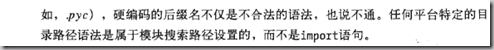Python语句

random模块高级用法：

 distributions on the real line: ------------------------       uniform        triangular        normal (Gaussian)        lognormal        negative exponential        gamma        beta        pareto        Weibull distributions on the circle (angles 0 to 2pi) ------------------------       circular uniform        von Mises uniform(self, a, b) |      Get a random number in the range [a, b) or [a, b]. triangular(self, low=0.0, high=1.0, mode=None) |      Triangular distribution. gauss(self, mu, sigma) |      Gaussian distribution. normalvariate(self, mu, sigma) |      Normal distribution. lognormvariate(self, mu, sigma) |      Log normal distribution. expovariate(self, lambd) |      Exponential distribution. gammavariate(self, alpha, beta) |      Gamma distribution.  Not the gamma function! betavariate(self, alpha, beta) |      Beta distribution. paretovariate(self, alpha) |      Pareto distribution.  weibullvariate(self, alpha, beta) |      Weibull distribution. vonmisesvariate(self, mu, kappa) |      Circular data distribution. internal state seed(self, a=None) |      Initialize internal state from hashable object. setstate(self, state) |      Restore internal state from object returned by getstate(). getstate(self) |      Return internal state; can be passed to setstate() later. jumpahead(self, n) |      Change the internal state to one that is likely far away |      from the current state.  This method will not be in Py3.x, |      so it is better to simply reseed.